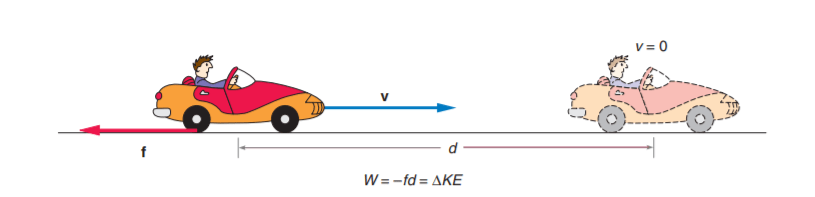# What is Negative Work? | Friction does negative work – How?

Last updated on January 24th, 2022 at 09:34 pm

A sign with a vector quantity denotes direction. But, Work is a scalar quantity. So a negative sign with it (i.e. a negative work) has no connection with its direction. Then what is negative work?

When work done by an external force on a mass reduces the energy of that mass then we say that the work done is negative. The energy here does not disappear, but is, instead, lost to the surroundings in the form of heat or thermal energy.

## Friction does negative work – How?

When an object moves the frictional force opposes the motion. The angle between the frictional force(F) and the displacement(s) is 180°.

[note that cos 180° = – 1]

So the work done on that object by the frictional force is expressed as W = Fs cos 180° = – Fs

Please note the negative sign. That sign denotes that the work done by the resistive force friction on the object in motion is negative as it reduces the energy (KE) of the object.

### Example of negative work

Suppose, for example, that we apply the brakes to a rapidly moving car and the car skids to a stop. Let’s see how the frictional force exerted by the road surface on the tires of the car does work.

When the car skids to a stop, it loses kinetic energy. A decrease in kinetic energy can be thought of as a negative change in kinetic energy.

If the change in kinetic energy is negative, the work done on the car should also be negative.figure 1: Frictional forces exerted on the car’s tires by the road surface do negative work in stopping the car, resulting in a decrease in kinetic energy.

Note that the frictional force exerted on the car acts in the opposite direction to the motion of the car shown in figure 1. When this is so, we say that the work done on the car by the force is negative work, removing energy from the system (the car) rather than increasing its energy.